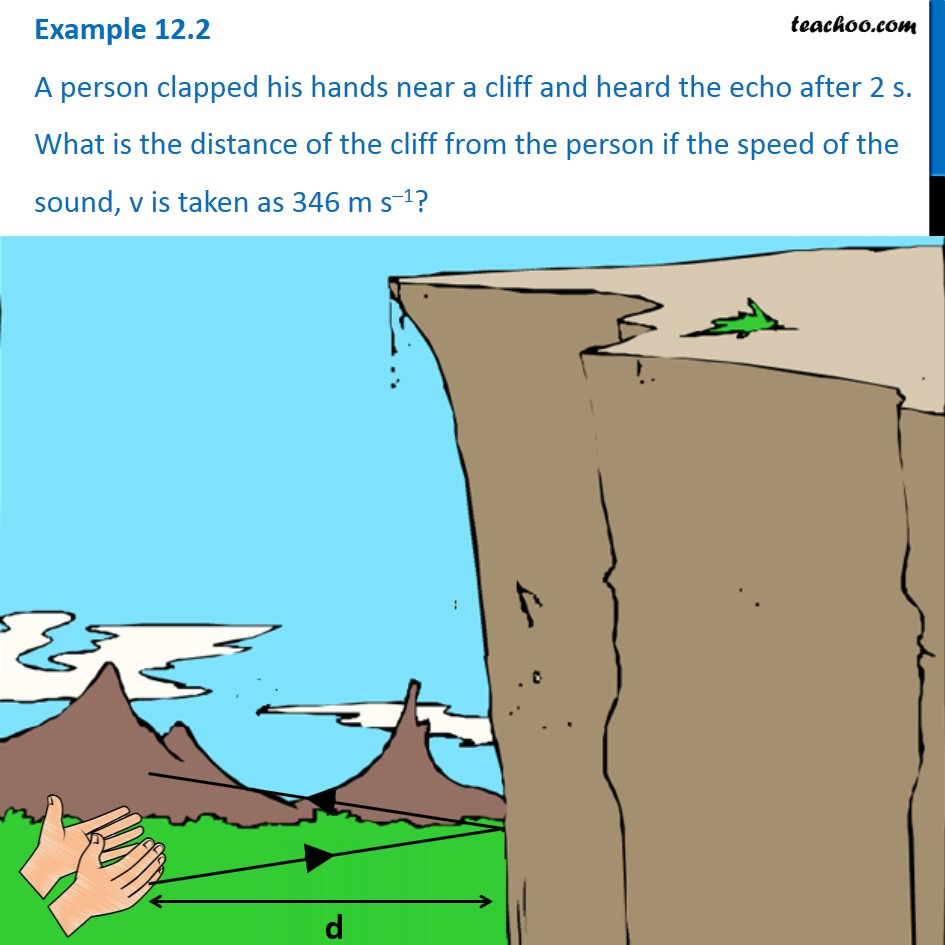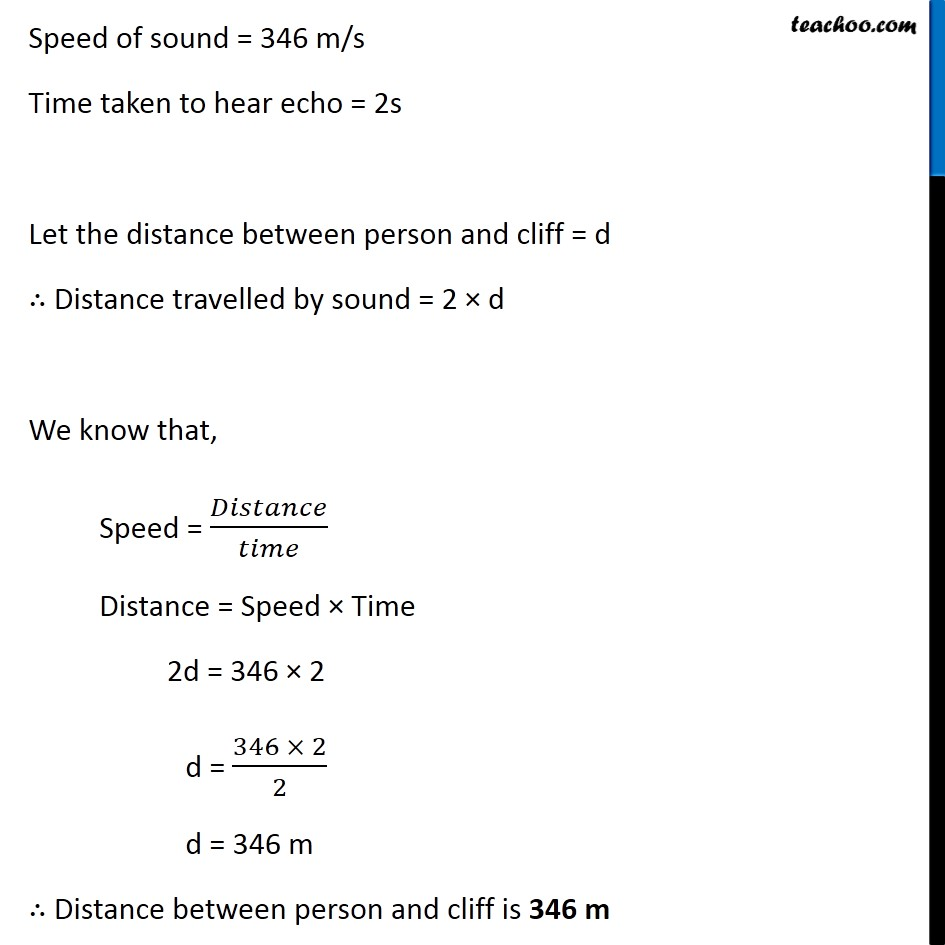Examples from NCERT Book

Class 9
Chapter 12 Class 9 - SoundLearn in your speed, with individual attention - Teachoo Maths 1-on-1 Class

### Transcript

Example 12.2 A person clapped his hands near a cliff and heard the echo after 2 s. What is the distance of the cliff from the person if the speed of the sound, v is taken as 346 m s–1? Speed of sound = 346 m/s Time taken to hear echo = 2s Let the distance between person and cliff = d ∴ Distance travelled by sound = 2 × d We know that, Speed = 𝐷𝑖𝑠𝑡𝑎𝑛𝑐𝑒/𝑡𝑖𝑚𝑒 Distance = Speed × Time 2d = 346 × 2 d = (346 × 2)/2 d = 346 m ∴ Distance between person and cliff is 346 m